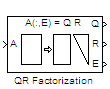# QR Factorization

Factor arbitrary matrix into unitary and upper triangular components

## Library

Math Functions / Matrices and Linear Algebra / Matrix Factorizations

`dspfactors`

•## Description

The QR Factorization block uses a sequence of Householder transformations to triangularize the input matrix A. The block factors a column permutation of the M-by-N input matrix A as

Ae = QR

The column-pivoted matrix Ae contains the columns of A permuted as indicated by the contents of length-N permutation vector E.

```Ae = A(:,E) % Equivalent MATLAB code ```

The block selects a column permutation vector E, which ensures that the diagonal elements of matrix R are arranged in order of decreasing magnitude.

The size of matrices Q and R depends on the setting of the Output size parameter:

• When you select `Economy` for the output size, Q is an M-by-min(M,N) unitary matrix, and R is a min(M,N)-by-N upper-triangular matrix.

`[Q R E] = qr(A,0) % Equivalent MATLAB code`

• When you select `Full` for the output size, Q is an M-by-M unitary matrix, and R is a M-by-N upper-triangular matrix.

`[Q R E] = qr(A) % Equivalent MATLAB code`

The block treats length-M unoriented vector input as an M-by-1 matrix.

QR factorization is an important tool for solving linear systems of equations because of good error propagation properties and the invertibility of unitary matrices:

Q –1 = Q`'`

where Q`'` is the complex conjugate transpose of Q.

Unlike LU and Cholesky factorizations, the matrix A does not need to be square for QR factorization. However, QR factorization requires twice as many operations as LU Factorization (Gaussian elimination).

## Parameters

Output size

Specify the size of output matrices Q and R:

• `Economy` — When this output size is selected, the block outputs an M-by-min(M,N) unitary matrix Q and a min(M,N)-by-N upper-triangular matrix R.

• `Full` — When this output size is selected, the block outputs an M-by-M unitary matrix Q and a M-by-N upper-triangular matrix R.

Simulate using

• `Interpreted execution` (default)

Simulate model using the MATLAB® interpreter. This option shortens startup time and has faster simulation speed compared to `Code generation`.

• `Code generation`

Simulate model using generated C code. The first time you run a simulation, Simulink® generates C code for the block. The C code is reused for subsequent simulations, as long as the model does not change. This option requires additional startup time but provides faster subsequent simulations.

## References

Golub, G. H., and C. F. Van Loan. Matrix Computations. 3rd ed. Baltimore, MD: Johns Hopkins University Press, 1996.

## Supported Data Types

PortSupported Data Types

Input

• Double-precision floating point

• Single-precision floating point

Output

• Double-precision floating point

• Single-precision floating point

## Version History

Introduced before R2006a